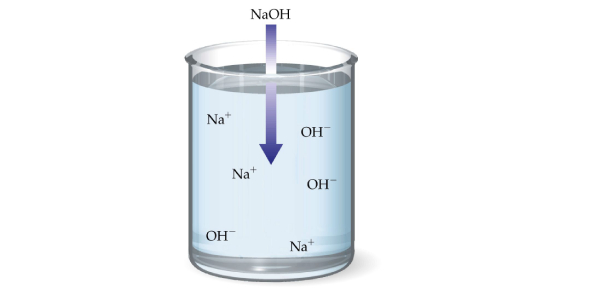What is the concentration of the acid if 20 ml is needed to titrate - ProProfs Discuss+ Ask Question

# What is the concentration of the acid if 20 ml is needed to titrate 35 mL of acid? The concentration of NaOH is 0.5 M.Change Image    Delete

A. 0.875 M of acid
B. 0.0029 M of the acid
C. 0.29 M of the acid
D. 0.00875 M of acid

This question is part of titration problems

Asked by Holmes, Last updated: Sep 27, 2020

###Request 0FollowShareAnswer AnonymouslyAnswer LaterCopy Link0.00875 m of acid is the concentration of the acidJohn Smith0.29 M of the acid
Since the name of the acid (and the equation) is not given, you must assume that it is a 1:1 ratio. Use equation: volume of acid x molarity of acid = volume of base x molarity of base (when the ratio is 1:1).Search for Google imagesSelect a recommended image
Upload from your computerCancelSearch for Google imagesSelect a recommended image
Upload from your computerCancelSearch for Google imagesSelect a recommended image
Upload from your computerCancel